Related Articles

# How to sum negative and positive values using GroupBy in Pandas?

• Last Updated : 30 May, 2021

In this article, we will discuss how to calculate the sum of all negative numbers and positive numbers in DataFrame using the GroupBy method in Pandas.

To use the groupby() method use the given below syntax.

Syntax: df.groupby(column_name)

### Stepwise Implementation

Step 1: Creating lambda functions to calculate positive-sum and negative-sum values.

```pos = lambda col : col[col > 0].sum()
neg = lambda col : col[col < 0].sum()```

Step 2: We will use the groupby() method and apply the lambda function to calculate the sum.

```d = df.groupby(df['Alphabet'])
print(d['Frequency'].agg([('negative_values', neg),
('positive_values', pos)
]))
print(d['Bandwidth'].agg([('negative_values', neg),
('positive_values', pos)
]))```

### Examples

Example 1:

Calculate the sum of all positive as well as negative values of a, b, c for both columns i.e., Frequency and bandwidth

## Python3

 `# Import Necessary Libraries``import` `pandas as pd``import` `numpy as np`` ` `# Creating a DataFrame with ``# random values``df ``=` `pd.DataFrame({``'Alphabet'``: [``'a'``, ``'b'``, ``'c'``, ``'c'``,``                                ``'a'``, ``'a'``, ``'c'``, ``'b'``],``                    ` `                   ``'Frequency'``: [``-``10``, ``29``, ``-``12``, ``-``190``,``                                 ``72``, ``-``98``, ``-``12``, ``0``],``                    ` `                   ``'BandWidth'``: [``10``, ``34``, ``23``, ``-``10``, ``-``87``,``                                 ``-``76``, ``365``, ``10``]})`` ` `print``(df)`` ` `# Group By dataframe on categorical``# values``d ``=` `df.groupby(df[``'Alphabet'``])`` ` `# creating lambda function to calculate``# positive as well as negative values``def` `pos(col): ``  ``return` `col[col > ``0``].``sum``()`` ` `def` `neg(col): ``  ``return` `col[col < ``0``].``sum``()`` ` ` ` `# Apply lambda function to particular ``# column``print``(d[``'Frequency'``].agg([(``'negative_values'``, neg),``                          ``(``'positive_values'``, pos)``                          ``]))`` ` `print``(d[``'Bandwidth'``].agg([(``'negative_values'``, neg),``                          ``(``'positive_values'``, pos)``                          ``]))`

Output: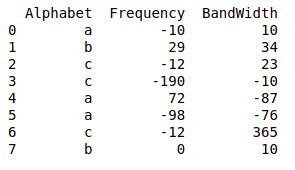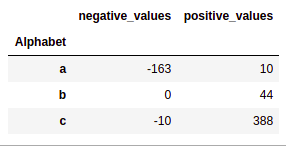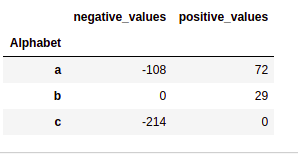Example 2:

Calculate the sum of all positive as well as negative values of a, b for both columns i.e., X and Y

## Python3

 `# Import Necessary Libraries``import` `pandas as pd``import` `numpy as np`` ` `# Creating a DataFrame with random values``df ``=` `pd.DataFrame({``'Function'``: [``'F(x)'``, ``'F(x)'``, ``'F(y)'``,``                                ``'F(x)'``, ``'F(y)'``, ``'F(x)'``,``                                ``'F(x)'``, ``'F(y)'``],``                    ` `                   ``'X'``: [``-``10``, ``29``, ``-``12``, ``-``190``, ``72``, ``-``98``,``                         ``-``12``, ``0``],``                    ` `                   ``'Y'``: [``10``, ``34``, ``23``, ``-``10``, ``-``87``, ``-``76``, ``                         ``365``, ``10``]})`` ` `print``(df)`` ` `# Group By dataframe on categorical values``d ``=` `df.groupby(df[``'Function'``])`` ` `# creating lambda function to calculate``# positive as well as negative values``def` `pos(col): ``  ``return` `col[col > ``0``].``sum``()`` ` `def` `neg(col): ``  ``return` `col[col < ``0``].``sum``()`` ` `# Apply lambda function to particular ``# column``print``(d[``'X'``].agg([(``'negative_values'``, neg),``                  ``(``'positive_values'``, pos)``                  ``]))`` ` `print``(d[``'Y'``].agg([(``'negative_values'``, neg),``                  ``(``'positive_values'``, pos)``                  ``]))`

Output: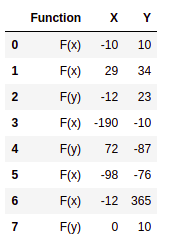DataFrame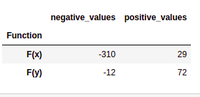X Output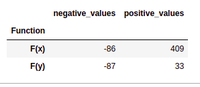Y Output

Example 3:

Calculate the sum of all positive as well as negative values of every name i.e., Marks. The next step is to make the lambda function to calculate the sum. In the last step, we will group the data according to the names and call the lambda functions to calculate the sum of the values.

## Python3

 `# Import Necessary Libraries``import` `pandas as pd``import` `numpy as np`` ` `# Creating a DataFrame with random values``df ``=` `pd.DataFrame({``'Name'``: [``'Aryan'``, ``'Nityaa'``, ``'Dhruv'``,``                            ``'Dhruv'``, ``'Nityaa'``, ``'Aryan'``,``                            ``'Nityaa'``, ``'Aryan'``, ``'Aryan'``, ``                            ``'Dhruv'``, ``'Nityaa'``, ``'Dhruv'``, ``                            ``'Dhruv'``],``                   ``'Marks'``: [``90``, ``93``, ``78``, ``56``, ``34``, ``12``, ``67``, ``                             ``45``, ``78``, ``92``, ``29``, ``88``, ``81``]})``print``(df)`` ` `# Group By dataframe on categorical values``d ``=` `df.groupby(df[``'Name'``])`` ` `# creating lambda function to calculate``# positive as well as negative values``def` `pos(col): ``  ``return` `col[col > ``0``].``sum``()`` ` `def` `neg(col): ``  ``return` `col[col < ``0``].``sum``()`` ` ` ` `# Apply lambda function to particular``# column``print``(d[``'Marks'``].agg([(``'negative_values'``, neg),``                      ``(``'positive_values'``, pos)``                      ``]))`

Output: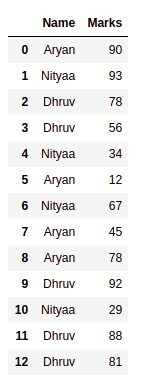Names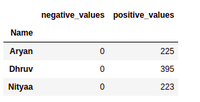Marks

Attention geek! Strengthen your foundations with the Python Programming Foundation Course and learn the basics.

To begin with, your interview preparations Enhance your Data Structures concepts with the Python DS Course. And to begin with your Machine Learning Journey, join the Machine Learning – Basic Level Course

My Personal Notes arrow_drop_up